# 2D density contour plots in ggplot2

## The `geom_density_2d` function

A 2D density contour plot can be created in ggplot2 with `geom_density_2d`. You just need to pass your data frame and indicate the `x` and `y` variable inside `aes`.

``````# install.packages("ggplot2")
library(ggplot2)

# Data
set.seed(1)
df <- data.frame(x = rnorm(200), y = rnorm(200))

ggplot(df, aes(x = x, y = y)) +
geom_density_2d()``````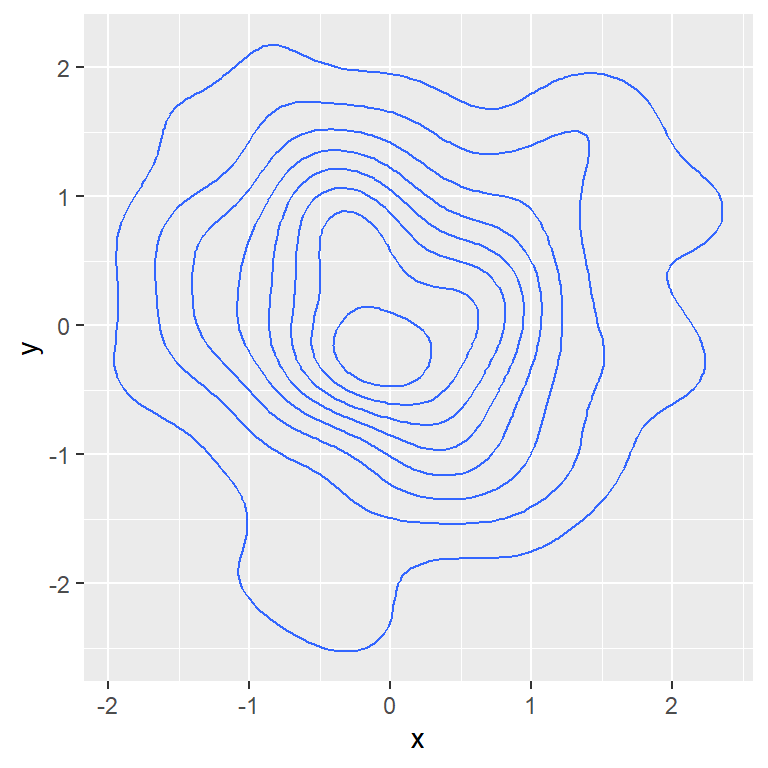Number of levels

It is possible to increase or decrease the number of levels with `bins`.

``````# install.packages("ggplot2")
library(ggplot2)

# Data
set.seed(1)
df <- data.frame(x = rnorm(200), y = rnorm(200))

ggplot(df, aes(x = x, y = y)) +
geom_density_2d(bins = 15)``````

Type `?geom_contour` for additional details.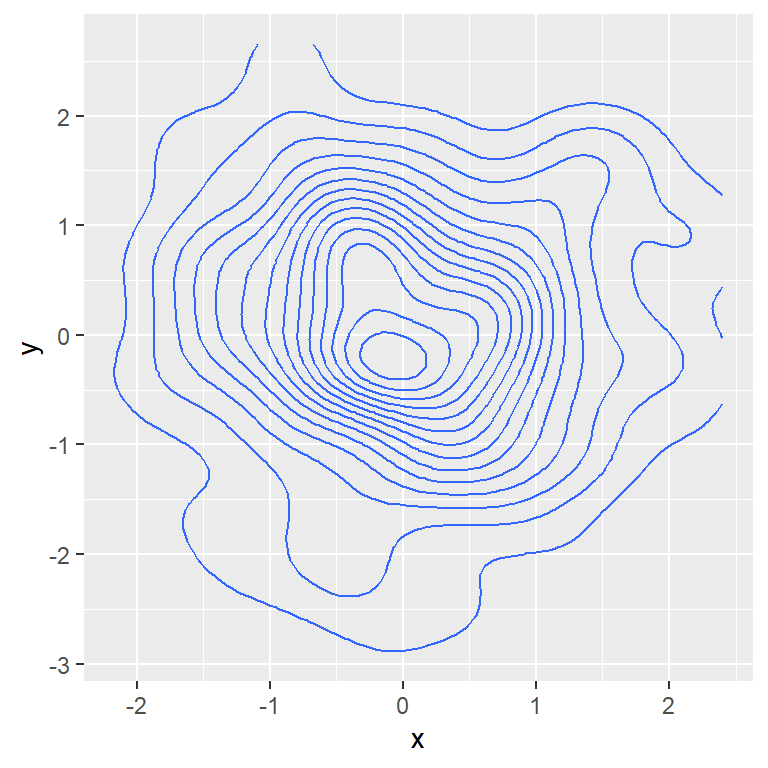Scatter plot with contour lines

Note that you can add the points with `geom_point` and then `geom_density_2d` to create a scatter plot with their corresponding contour lines.

``````# install.packages("ggplot2")
library(ggplot2)

# Data
set.seed(1)
df <- data.frame(x = rnorm(200), y = rnorm(200))

ggplot(df, aes(x = x, y = y)) +
geom_point() +
geom_density_2d()``````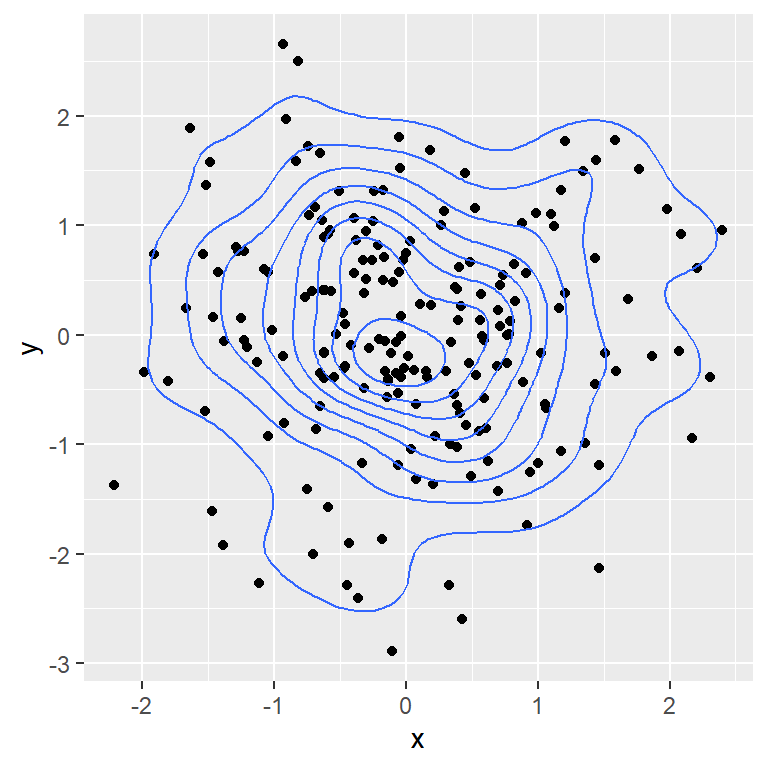Color

You can customize the lines in several ways, changing the color, line type of size of the lines, as in other plots.

``````# install.packages("ggplot2")
library(ggplot2)

# Data
set.seed(1)
df <- data.frame(x = rnorm(200), y = rnorm(200))

ggplot(df, aes(x = x, y = y)) +
geom_density_2d(color = "red")``````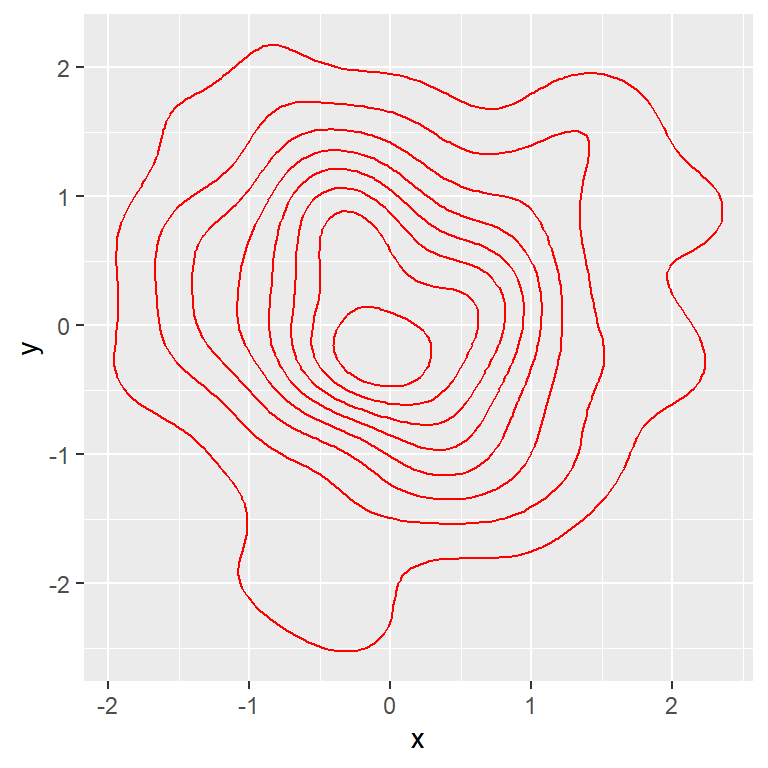Color based on the level

Passing `..level..` to the `color` argument of the `aes` will colorize each contour line based on the level. This will highlight the areas with higher density.

``````# install.packages("ggplot2")
library(ggplot2)

# Data
set.seed(1)
df <- data.frame(x = rnorm(200), y = rnorm(200))

ggplot(df, aes(x = x, y = y)) +
geom_density_2d(aes(color = ..level..))``````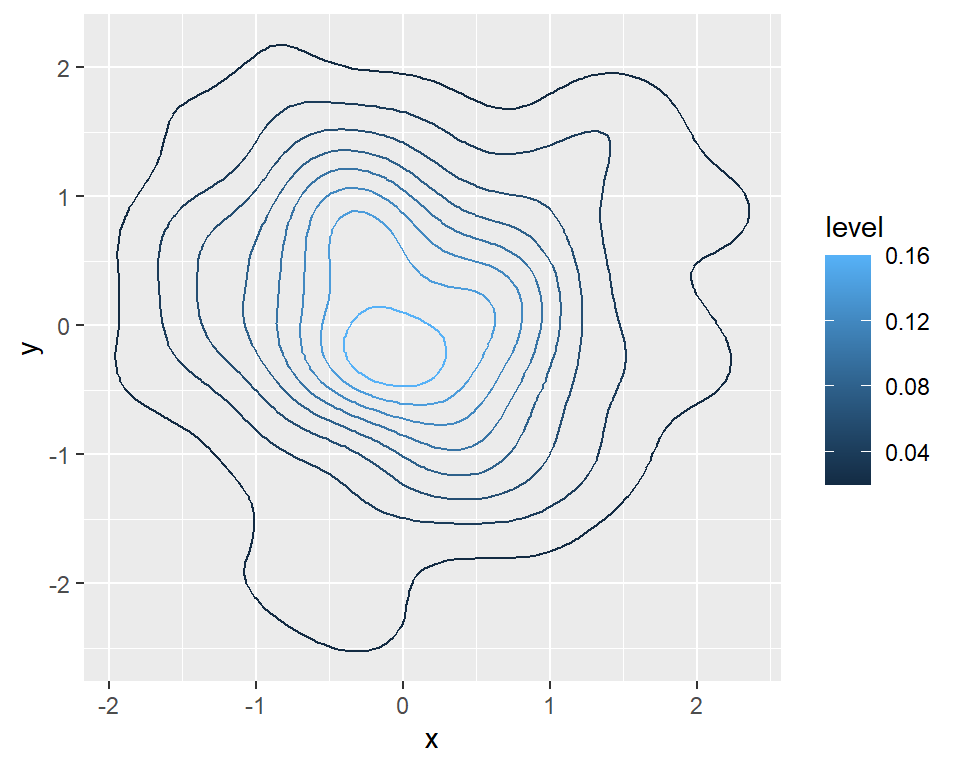Change the color palette

If the lines are colored based on the level you can change the color palette with a continuous color palette. For instance, you can pass the `scale_color_viridis_c` to use the viridis palette.

``````# install.packages("ggplot2")
library(ggplot2)

# Data
set.seed(1)
df <- data.frame(x = rnorm(200), y = rnorm(200))

ggplot(df, aes(x = x, y = y)) +
geom_density_2d(aes(color = ..level..)) +
scale_color_viridis_c()``````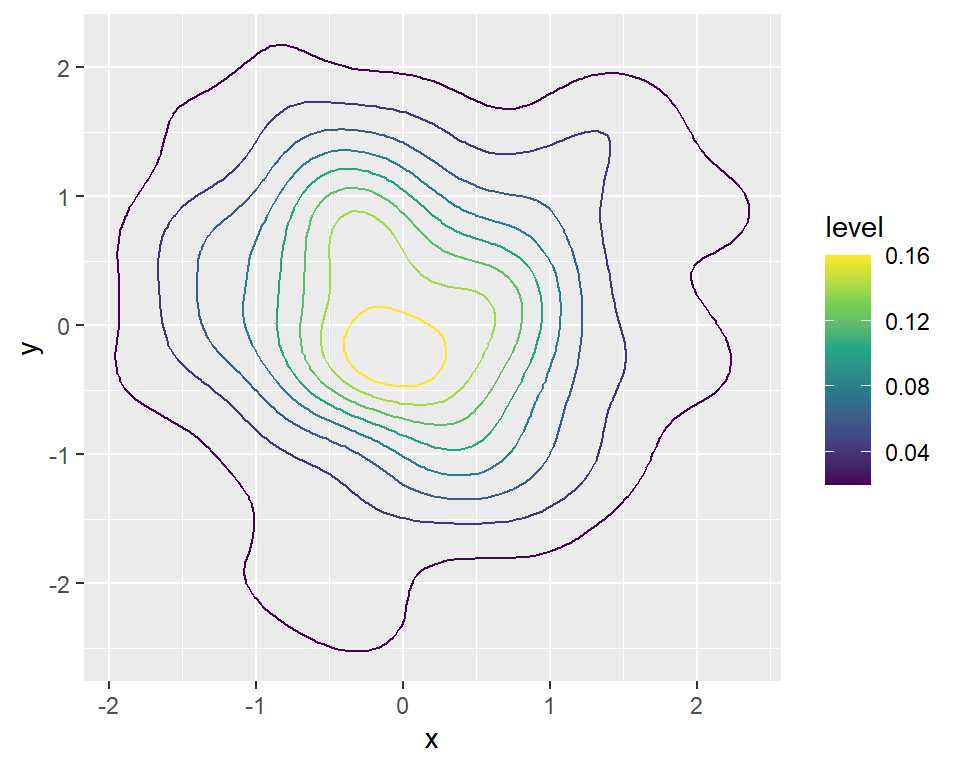Fill the contour with stat_density_2d

Note that there is a similar function named `stat_density_2d` that allows changing the geom used. If you set `geom = "polygon"` you can fill the contour as follows. Alternatively you can use `geom_density_2d_filled`.

``````# install.packages("ggplot2")
library(ggplot2)

# Data
set.seed(1)
df <- data.frame(x = rnorm(200), y = rnorm(200))

ggplot(df, aes(x = x, y = y, fill = ..level..)) +
stat_density_2d(geom = "polygon")``````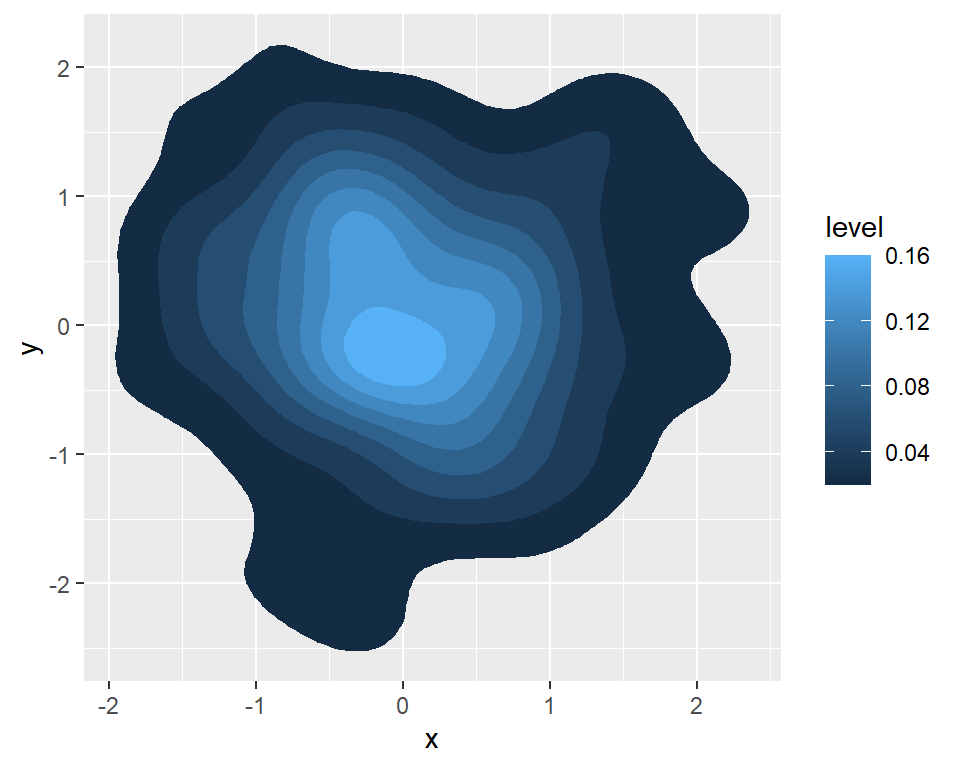## The `geom_density_2d_filled` function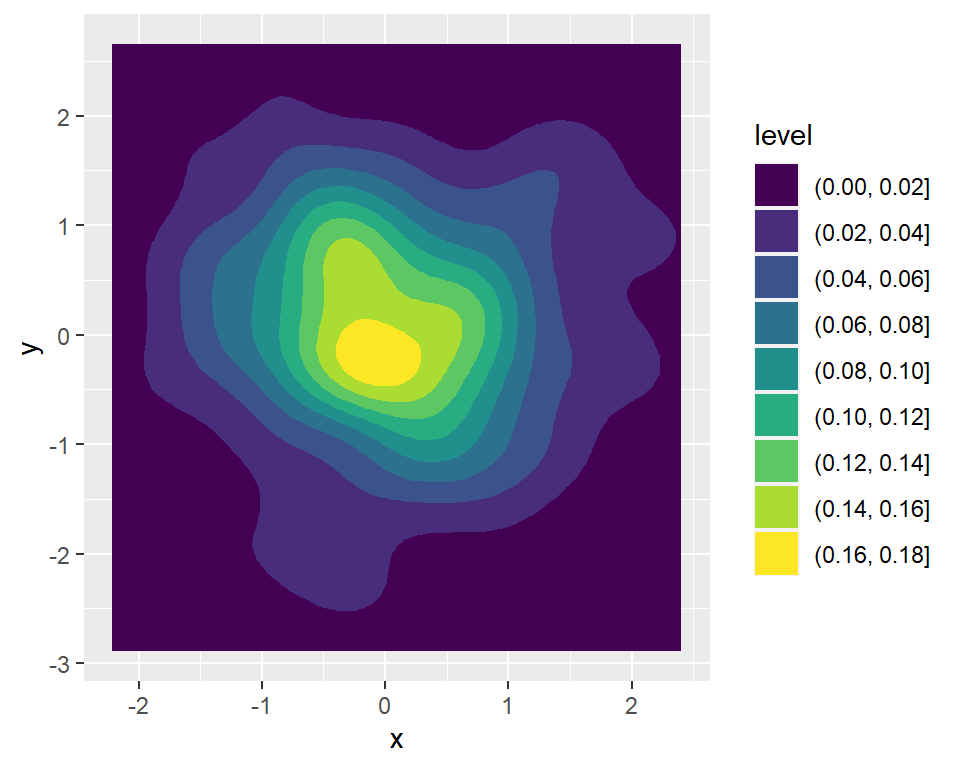If you want to fill the areas of the contour based on the level you can use the `geom_density_2d_filled` function.

``````# install.packages("ggplot2")
library(ggplot2)

# Data
set.seed(1)
df <- data.frame(x = rnorm(200), y = rnorm(200))

ggplot(df, aes(x = x, y = y)) +
geom_density_2d_filled()``````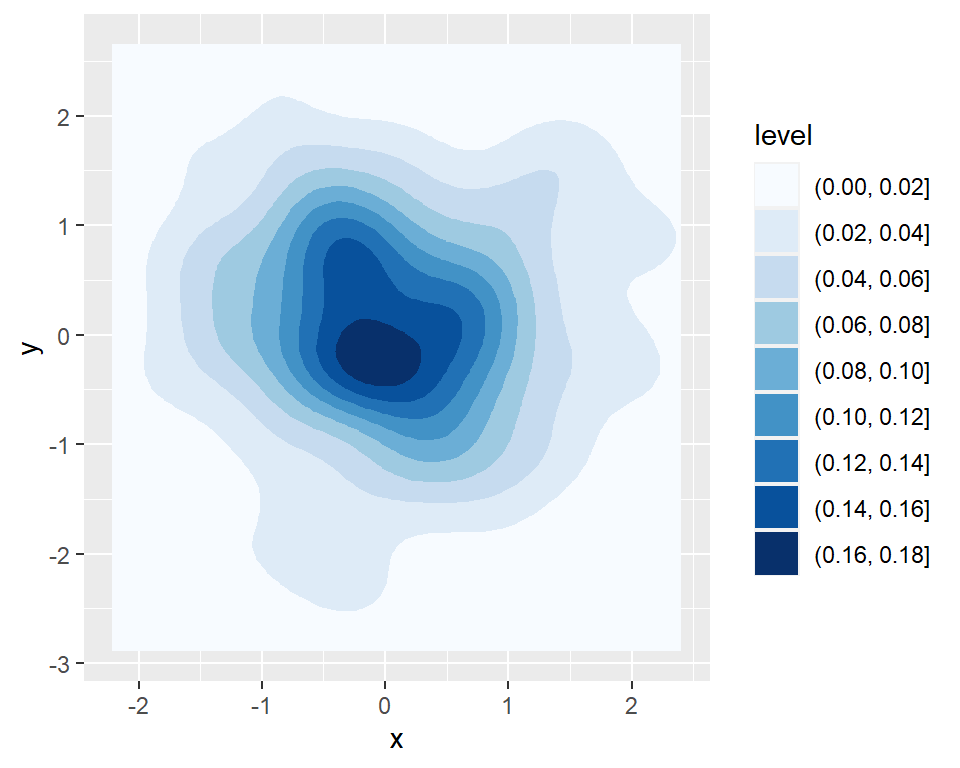Color palette

You can change the color palette with a fill scale, such as the `scale_fill_brewer` or `scale_fill_manual` for custom colors.

``````# install.packages("ggplot2")
library(ggplot2)

# Data
set.seed(1)
df <- data.frame(x = rnorm(200), y = rnorm(200))

ggplot(df, aes(x = x, y = y)) +
geom_density_2d_filled() +
scale_fill_brewer()``````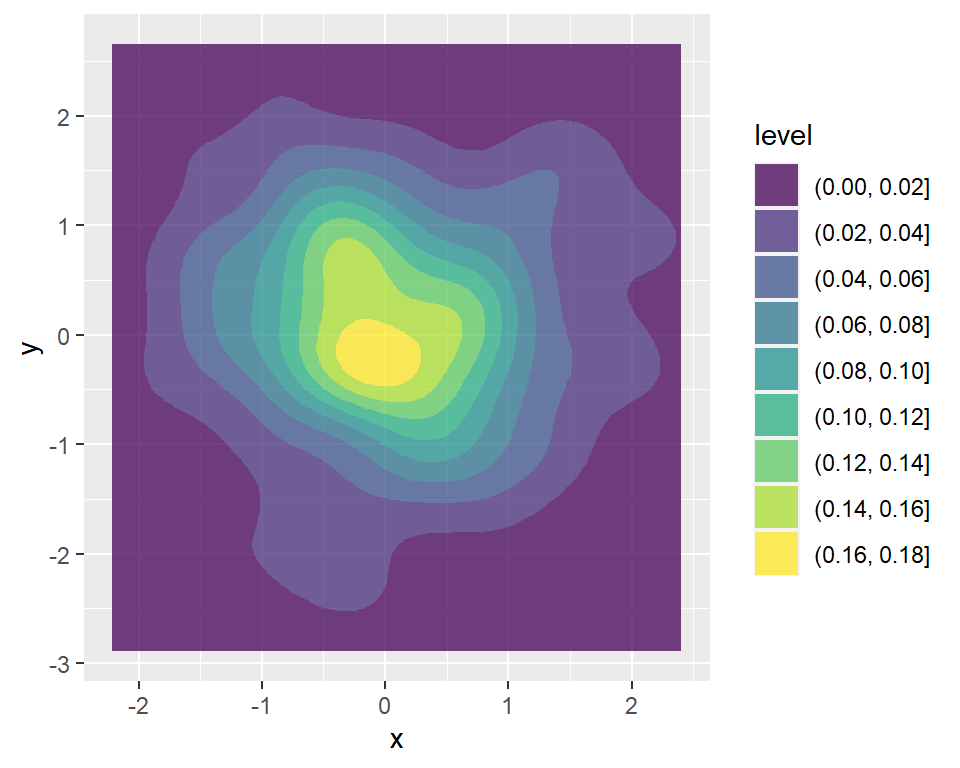Transparency

The `alpha` argument controls the transparency of the filled areas.

``````# install.packages("ggplot2")
library(ggplot2)

# Data
set.seed(1)
df <- data.frame(x = rnorm(200), y = rnorm(200))

ggplot(df, aes(x = x, y = y)) +
geom_density_2d_filled(alpha = 0.75)``````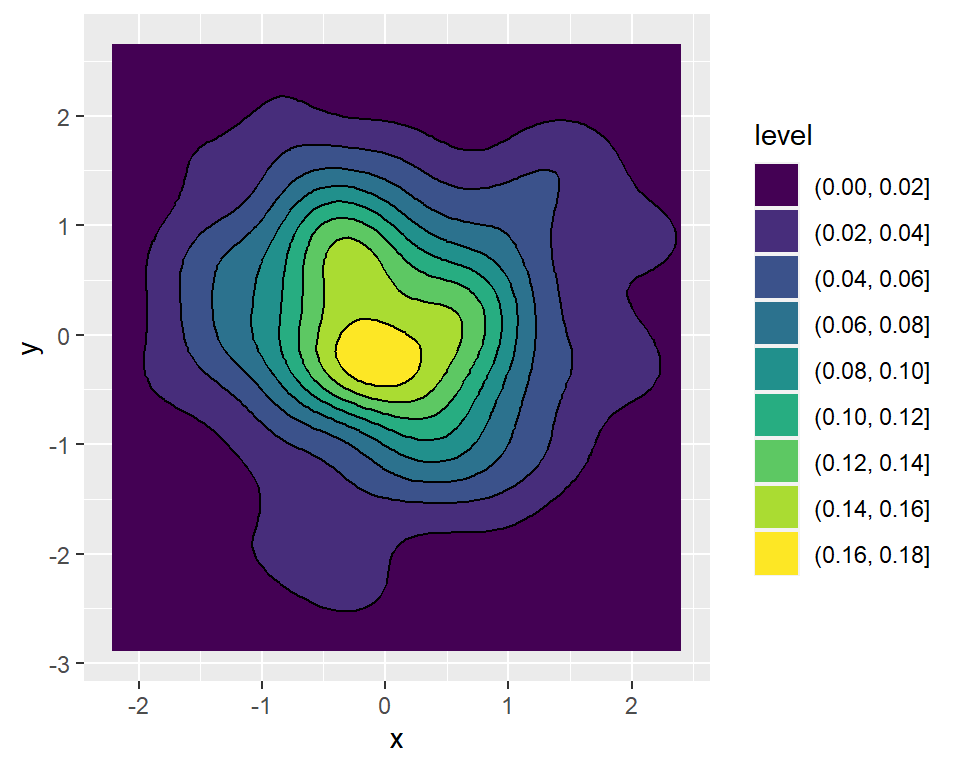Filled contour with lines

Note that you can also add the contour lines over the filled contour.

``````# install.packages("ggplot2")
library(ggplot2)

# Data
set.seed(1)
df <- data.frame(x = rnorm(200), y = rnorm(200))

ggplot(df, aes(x = x, y = y)) +
geom_density_2d_filled() +
geom_density_2d(colour = "black")``````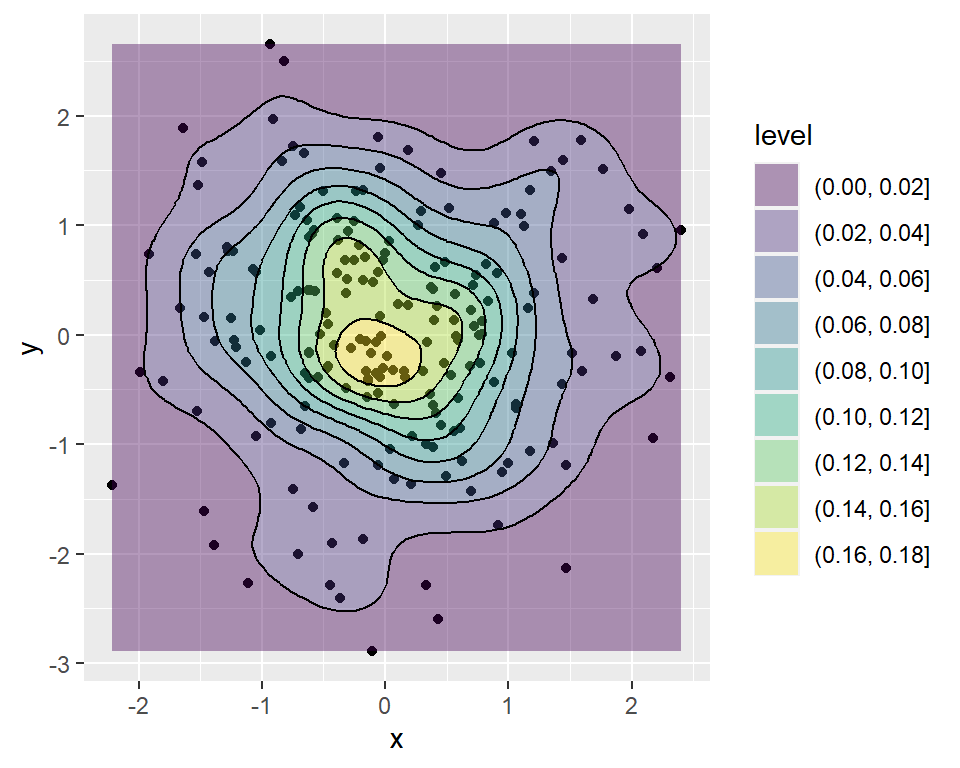Points and filled contour

It is worth to mention that you can add a filled contour over a scatter plot, setting a low transparency.

``````# install.packages("ggplot2")
library(ggplot2)

# Data
set.seed(1)
df <- data.frame(x = rnorm(200), y = rnorm(200))

ggplot(df, aes(x = x, y = y)) +
geom_point() +
geom_density_2d_filled(alpha = 0.4) +
geom_density_2d(colour = "black")``````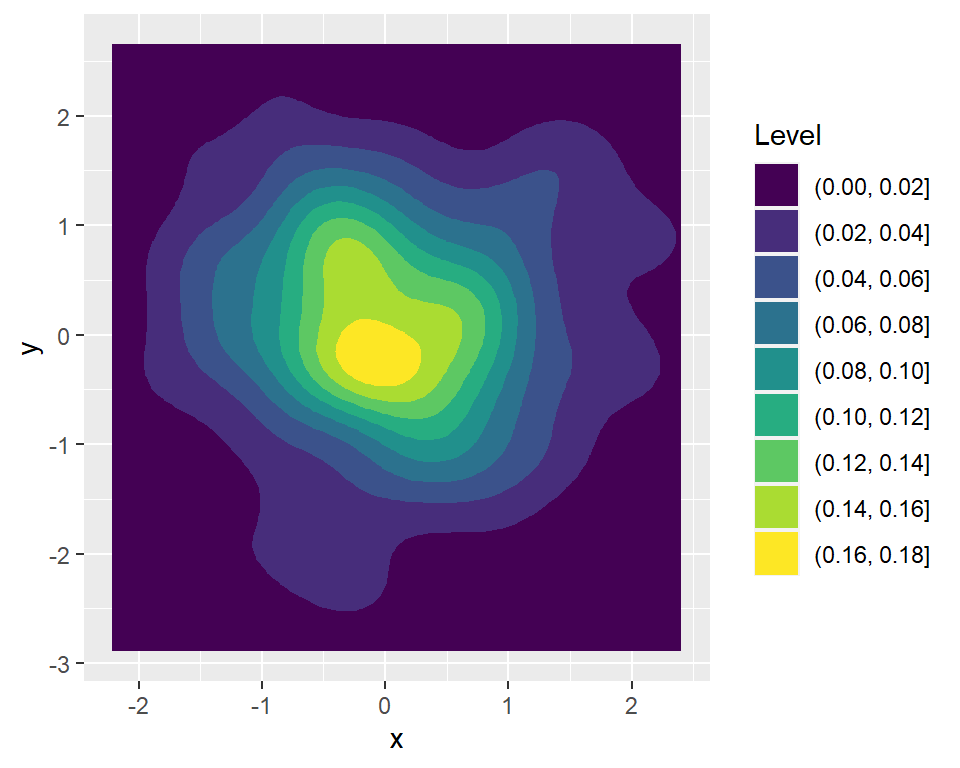Title of the legend

The legend of the contour plots can be customized. In the following example we are changing the title of the legend.

``````# install.packages("ggplot2")
library(ggplot2)

# Data
set.seed(1)
df <- data.frame(x = rnorm(200), y = rnorm(200))

ggplot(df, aes(x = x, y = y)) +
geom_density_2d_filled() +
guides(fill = guide_legend(title = "Level"))``````